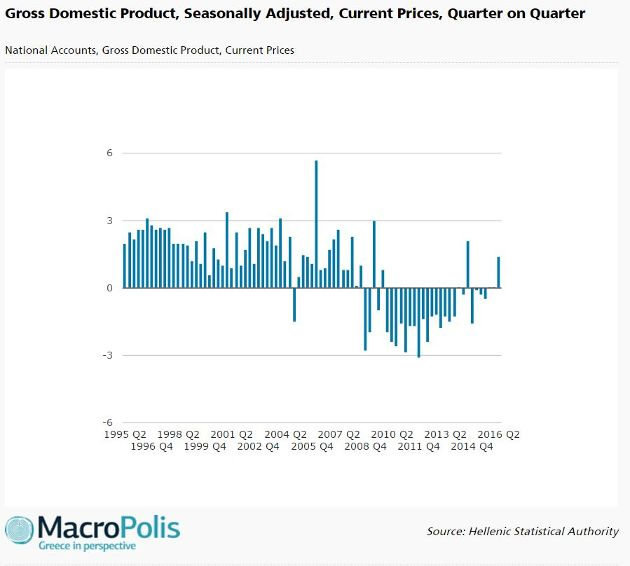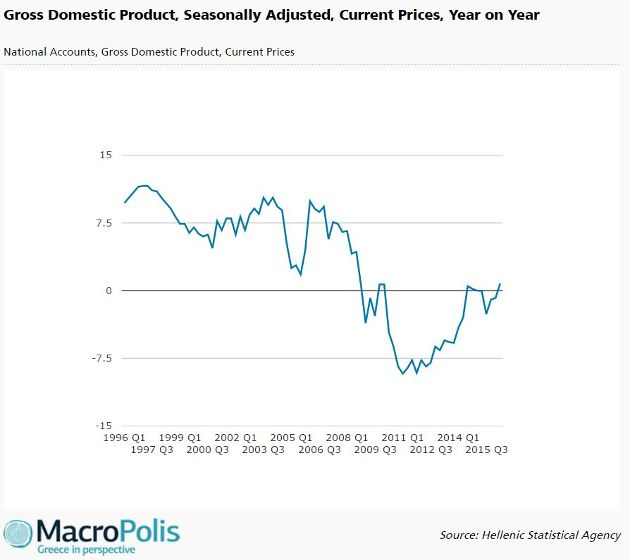Greece's latest GDP figures and what to expect for the rest of the year

Agora Contributor: Manos Giakoumis

The flash estimates released by the Hellenic Statistical Authority (ELSTAT) on Friday were a positive surprise, with the seasonally-adjusted (SA) GDP growing by 0.3 percent quarter on quarter (QoQ) in the second quarter (Q2) of 2016, while it contracted by 0.7 percent year on year (YoY).

It should be noted that consensus estimates and Reuters poll were pointing to a 0.2 percent drop and 0.1 percent rise respectively QoQ in Q2 GDP.

ELSTAT also revised upward the previous quarters’ GDP. The most notable revisions were for Q4 2015 and Q1 2016, which now stand at 0.2 and 0.6 percent, respectively, above previous levels. In absolute terms, the past two quarters GDP were upgraded by 92 and 282 million euros respectively.

Taking also into account the 0.3 percent QoQ increase in Q2, we now have a higher Q2 GDP (and consequently H1) figure in absolute terms versus earlier estimates.Although detailed figures and a potential revision of Q2 GDP are due upon the release of provisional data on August 29, there are some interesting conclusions that can be drawn from ELSTAT flash estimates.

In absolute terms, Q2 GDP of 46.19 billion euros is the highest reading since capital controls were introduced (i.e. in the last four quarters).

Another development, not widely noticed, is the impressive 1.4 percent QoQ rise in Q2 nominal GDP after it either remained stable (in the previous two quarters) or dropped (in the preceding four quarters) in the last six quarters.This explains why Q2 nominal GDP also grew by 0.8 percent YoY, which is the first positive reading since Q4 2014 and the highest since Q3 2008.

Overall, nominal GDP remained stable in the first half of the year, in contrast to real GDP, which contracted 0.7 percent.Moreover, the QoQ increase of 0.3 percent in real GDP and 1.4 percent in nominal GDP in Q2 2016 are both the highest readings since Q3 2014, when GDP grew by 1.4 and 2.1 percent respectively.

A logical question after the announcement of Q2 figures is whether the full year forecasts can be met.

The Bank of Greece (BoG) and the European Commission (EC) are expecting a mild recession of 0.3 percent for 2016, the OECD a slightly lower GDP contraction of 0.2 percent, while the rating agencies are more pessimistic. Moody’s and S&P forecast a recession of 0.7 and 1 percent respectively.

Assuming in absolute terms SA GDP remains at Q2 levels over the next two quarters, Q3 and Q4 GDP would increase by 0.4 and 0.2 percent respectively leading 2016 GDP down by 0.2 percent. This means it is reasonable to conclude that the 0.2-0.3 percent recession projected by the BoG, EC and the OECD is now in sight.

It is worth remembering that GDP contracted by 1.1 percent QoQ in Q3 2015 in the wake of capital controls but rebounded by only a marginal 0.2 percent in Q4 2015. As a result, GDP in the second half of 2015 (92.09 billion euros) was 0.9 percent lower than that in the first half (92.95 billion), meaning the second half of 2016 is benefitting from a low base effect.

Τhis explains the anticipated YoY rise in GDP in Q3 and Q4 2016, after four quarters of YoY decline ranging between 0.7 to 1.7 percent, assuming that GDP remains at Q2 levels in the next two quarters.

If GDP reaches 46.4 billion euros in Q3 and Q4, corresponding to a rebound of 0.5 percent from Q2 level, 2016 would end up with flat growth. In contrast, if GDP falls back to its Q1 level in the next two quarters, then the Greek economy would contract by 0.4 percent in 2016.

1 Comment(s)

• Posted by: Dean Plassaras